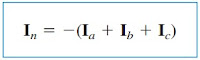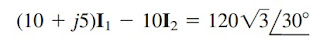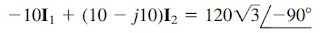omG8HI9LSKFW6lhCj8prTs0Z6lUhdOT9Jhi1Sf4m

# Unbalanced Three-Phase Systems Full Analysis

A special technique for handling unbalanced three-phase systems is the method of symmetrical components, which is beyond the scope of this text

Make sure to read what is three-phase circuit first.

This chapter would be incomplete without mentioning unbalanced three-phase systems. An unbalanced system is caused by two possible situations: (1) the source voltages are not equal in magnitude and/or differ in phase by angles that are unequal, or (2) load impedances are unequal.
Thus,
An unbalanced system is due to unbalanced voltage sources or an unbalanced load.
To simplify the analysis, we will assume balanced source voltages, but an unbalanced load.

Unbalanced three-phase systems are solved by the direct application of mesh and nodal analysis. Figure.(1) shows an example of an unbalanced three-phase system that consists of balanced source voltages (not shown in the figure) and an unbalanced Y-connected load (shown in the figure).Figure 1. Unbalanced three-phase Y-connected load
Since the load is unbalanced, ZAZB, and ZC are not equal. The line currents are determined by Ohm’s law as(1)
This set of unbalanced line currents produces current in the neutral line, which is not zero as in a balanced system. Applying KCL at node N gives the neutral line current as(2)
In a three-wire system where the neutral line is absent, we can still find the line currents IaIb, and Ic using mesh analysis. A node N, KCL must be satisfied so that Ia + Ib + Ic = 0 in this case. The same could be done for a ∆-Y, Y-∆, or ∆-∆ three-wire system.

As mentioned earlier, in long-distance power transmission, conductors in multiples of three (multiple three-wire systems) are used, with the earth itself acting as the neutral conductor.

To calculate power in an unbalanced three-phase system requires that we find the power in each phase. The total power is not simply three times the power in one phase but the sum of the powers in the three phases.

## Unbalanced Three-Phase Systems Examples

1. The unbalanced Y-load of Figure.(1) has balanced voltages of 100 V and the a c b sequence. Calculate the line currents and the neutral current. Take ZA = 15 Ω , ZB = 10 + j5 Ω, ZC = 6 − j8 Ω.
Solution:
Using Equation.(1), the line currents are
Using Equation.(2), the current in the neutral line is

2. For the unbalanced circuit in Figure.(2), find (a) the line currents, (b) the total complex power absorbed by the load, and (c) the total complex power supplied by the source.Figure 2
Solution:
(a) We use mesh analysis to find the required currents. For mesh 1,
or(2.1)
For mesh 2,
or(2.2)
Equations.(2.1) and (2.2) form a matrix equation:
The determinants are
The mesh currents are
The line currents are

(b) We can now calculate the complex power absorbed by the load. For phase A,
For phase B,
For phase C,
The total complex power absorbed by the load is

(c) We check the result above by finding the power supplied by the source. For the voltage source in phase a,
For the source in phase b,
For the source in phase c,
The total complex power supplied by the three-phase source is
showing that Ss + SL = 0 and confirming the conservation principle of ac power.

Have you understood what is unbalanced three-phase system? Don't forget to share and subscribe! Happy learning!
Reference:  Fundamentals of electric circuits by Charles K. Alexander and Matthew N. O. Sadiku
Related Posts
SHARE